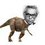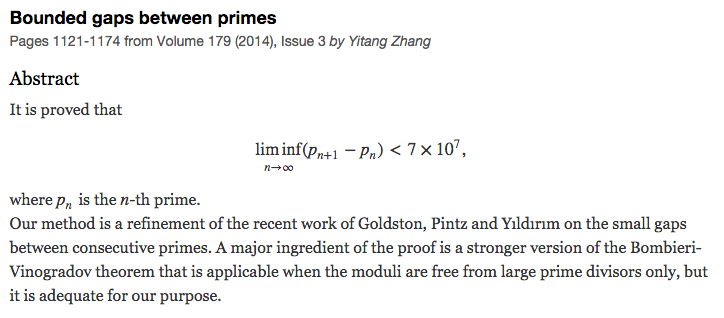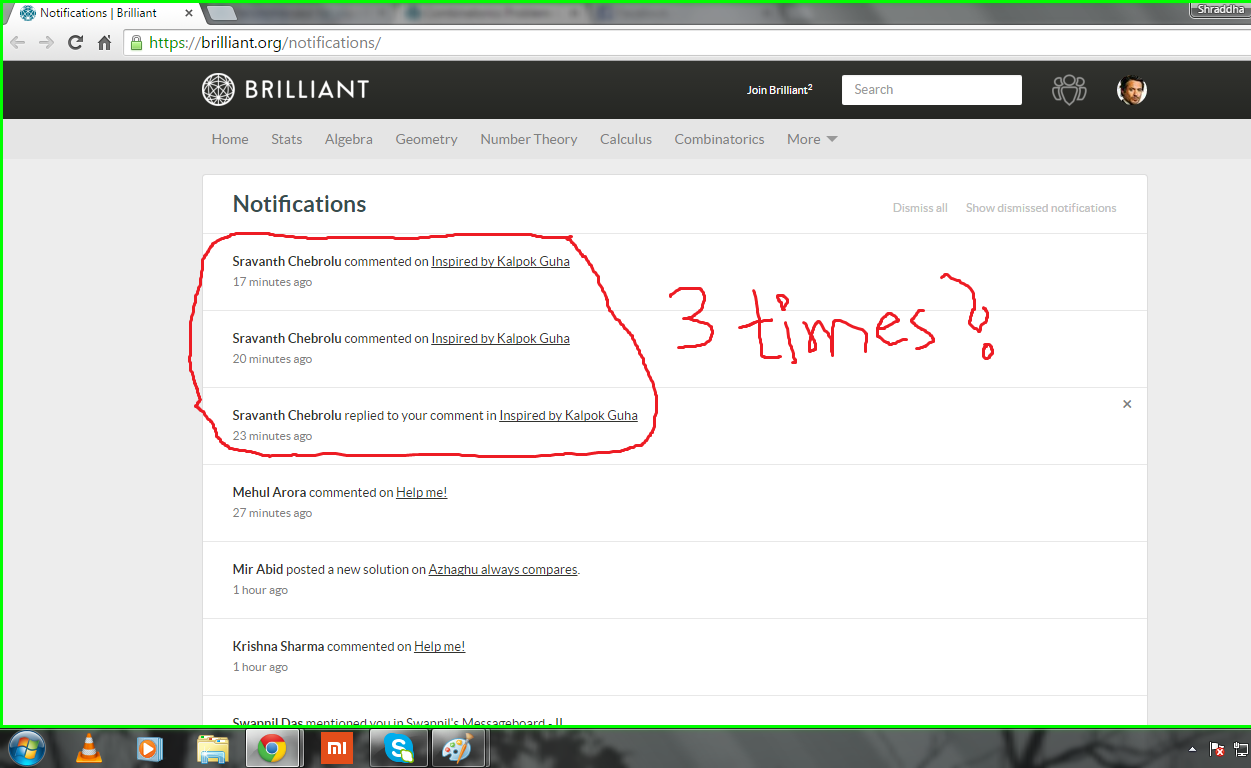# Inspired by Kalpok Guha

I happened to see this problem. So I came up with a similar problem below.

How many integers $n>1$ are there such that $n,n+2,n+6$ are all prime numbers ?

Do post Awesome solutions with proofs!Note by Nihar Mahajan
6 years, 2 months ago

This discussion board is a place to discuss our Daily Challenges and the math and science related to those challenges. Explanations are more than just a solution — they should explain the steps and thinking strategies that you used to obtain the solution. Comments should further the discussion of math and science.

When posting on Brilliant:

• Use the emojis to react to an explanation, whether you're congratulating a job well done , or just really confused .
• Ask specific questions about the challenge or the steps in somebody's explanation. Well-posed questions can add a lot to the discussion, but posting "I don't understand!" doesn't help anyone.
• Try to contribute something new to the discussion, whether it is an extension, generalization or other idea related to the challenge.

MarkdownAppears as
*italics* or _italics_ italics
**bold** or __bold__ bold
- bulleted- list
• bulleted
• list
1. numbered2. list
1. numbered
2. list
Note: you must add a full line of space before and after lists for them to show up correctly
paragraph 1paragraph 2

paragraph 1

paragraph 2

[example link](https://brilliant.org)example link
> This is a quote
This is a quote
    # I indented these lines
# 4 spaces, and now they show
# up as a code block.

print "hello world"
# I indented these lines
# 4 spaces, and now they show
# up as a code block.

print "hello world"
MathAppears as
Remember to wrap math in $$ ... $$ or $ ... $ to ensure proper formatting.
2 \times 3 $2 \times 3$
2^{34} $2^{34}$
a_{i-1} $a_{i-1}$
\frac{2}{3} $\frac{2}{3}$
\sqrt{2} $\sqrt{2}$
\sum_{i=1}^3 $\sum_{i=1}^3$
\sin \theta $\sin \theta$
\boxed{123} $\boxed{123}$

Sort by:

- 6 years, 2 months ago

My intuition says there are infinitely many such $n$.

- 6 years, 2 months ago

If there are infinitely many such n, then the twin prime conjecture is true. I suggest working on the twin prime conjecture first, because it is (likely) easier.

Staff - 6 years, 2 months ago

Yeah , I also think that.Thanks!

- 6 years, 1 month ago

Has anyone come close to proving the twin prime conjecture or do we need to solve the Riemann hypothesis first?

- 6 years, 1 month ago

Zhang Yitang has proved a weakened form of the Twin Prime conjecture, namely that there exists an N such that there are infinitely many pairs of consecutive primes with difference < N.Here is a good introductory read to the relationships between TP and RH. It is written by Dan Goldston (same as the above), though prior to Zhang's discovery. If you can understand through the first 5 chapters, that would be great

The conjecture that the distribution of twin primes satisfies a Riemann Hypothesis type error term is well supported empirically, but I think this might be a problem that survives the current millennium.

Staff - 6 years, 1 month ago

Yes, even as I was working out I found many numbers,

Here's my explanation,

• First take prime numbers ending with $1$,

you can find that there can be many triplets satisfying the condition. For example: $\boxed{ 11, 13, 17}$, $\boxed{41,43,47}$, $\boxed{101,103,107}$ etc. . .

• Now, let's take primes ending with $2$,

As there is only one possibility, but that proves to be wrong. $\boxed{2,4,8}$

• Now, let's take primes ending with $3$,

We can say there's only no such possibility, because the second number i.e. $n+2$ yields us a number divisible by $5$. Even this triplet proves wrong, $\boxed{3,5,9}$ as $9$ is not prime.

• Now let's take primes ending with $5$,

Only one possibility, i.e. $\boxed{5,7,11}$. In all other triplets, the first number i.e. $n$ is not prime

• Now, let's take primes ending with $7$,

You can find that the can be many possibilities. For example: $\boxed{ 17, 19, 23}$, $\boxed{107,109,113}$, $\boxed{227,229,233}$ etc. . .

• Now, let's take primes ending with $9$,

No such possibility, because the third number i.e. $n+6$ yields us a number divisivisible by $5$

- 6 years, 2 months ago

I didn't know 71+6=79. :P

- 6 years, 2 months ago

@Sravanth Chebrolu I think you must develop a habit of always cross checking your work. :)

- 6 years, 2 months ago

Lol Btw Nice observation.

- 6 years, 2 months ago

197+6=203

- 6 years, 2 months ago

Where did you comment the 3 comments? I can only see one here.pic

- 6 years, 2 months ago

Ah! Those were deleted by me :P

- 6 years, 2 months ago

I guess U r the fastest one to get 200k points. Keep solving and making more problems.

- 6 years, 2 months ago

Thanks! I'll be posting a new set keep your eyes on that!

- 6 years, 2 months ago